• +91 9971497814
• info@interviewmaterial.com

# Chapter 15 Statistics Ex-15.3 Interview Questions Answers

### Related Subjects

Question 1 : From the data given below state which group is more variable, A or B?
 Marks 10 – 20 20 – 30 30 – 40 40 – 50 50 – 60 60 – 70 70 – 80 Group A 9 17 32 33 40 10 9 Group B 10 20 30 25 43 15 7

each series. Theseries having greater C.V. is said to be more variable than the other. Theseries having lesser C.V. is said to be more consistent than the other.

Co-efficient ofvariation (C.V.) = (σ/ x̅) × 100

Where, σ = standarddeviation, x̅ = mean

For Group A

 Marks Group A fi Mid-point Xi Yi = (xi – A)/h (Yi)2 fiyi fi(yi)2 10 – 20 9 15 ((15 – 45)/10) = -3 (-3)2 = 9 – 27 81 20 – 30 17 25 ((25 – 45)/10) = -2 (-2)2 = 4 – 34 68 30 – 40 32 35 ((35 – 45)/10) = – 1 (-1)2 = 1 – 32 32 40 – 50 33 45 ((45 – 45)/10) = 0 02 0 0 50 – 60 40 55 ((55 – 45)/10) = 1 12 = 1 40 40 60 – 70 10 65 ((65 – 45)/10) = 2 22 = 4 20 40 70 – 80 9 75 ((75 – 45)/10) = 3 32 = 9 27 81 Total 150 -6 342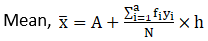Where A = 45,

and yi =(xi – A)/h

Here h = class size =20 – 10

h = 10

So, x̅ = 45 +((-6/150) × 10)

= 45 – 0.4

= 44.6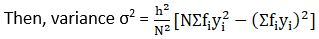σ2 =(102/1502) [150(342) – (-6)2]

= (100/22500) [51,300– 36]

= (100/22500) × 51264

= 227.84

Hence, standarddeviation = σ = √227.84

= 15.09

C.V for group A= (σ/ x̅) × 100

= (15.09/44.6) × 100

= 33.83

Now, for group B.

 Marks Group B fi Mid-point Xi Yi = (xi – A)/h (Yi)2 fiyi fi(yi)2 10 – 20 10 15 ((15 – 45)/10) = -3 (-3)2 = 9 – 30 90 20 – 30 20 25 ((25 – 45)/10) = -2 (-2)2 = 4 – 40 80 30 – 40 30 35 ((35 – 45)/10) = – 1 (-1)2 = 1 – 30 30 40 – 50 25 45 ((45 – 45)/10) = 0 02 0 0 50 – 60 43 55 ((55 – 45)/10) = 1 12 = 1 43 43 60 – 70 15 65 ((65 – 45)/10) = 2 22 = 4 30 60 70 – 80 7 75 ((75 – 45)/10) = 3 32 = 9 21 63 Total 150 -6 366Where A = 45,

h = 10

So, x̅ = 45 +((-6/150) × 10)

= 45 – 0.4

= 44.6σ2 =(102/1502) [150(366) – (-6)2]

= (100/22500) [54,900– 36]

= (100/22500) × 54,864

= 243.84

Hence, standarddeviation = σ = √243.84

= 15.61

C.V for group B= (σ/ x̅) × 100

= (15.61/44.6) × 100

= 35

By comparing C.V. ofgroup A and group B.

C.V of Group B >C.V. of Group A

So, Group B is morevariable.

Question 2 : From the prices of shares X and Y below, find out which is more stable in value:
 X 35 54 52 53 56 58 52 50 51 49 Y 108 107 105 105 106 107 104 103 104 101

From the given data,

Let us make the tableof the given data and append other columns after calculations.

 X (xi) Y (yi) Xi2 Yi2 35 108 1225 11664 54 107 2916 11449 52 105 2704 11025 53 105 2809 11025 56 106 8136 11236 58 107 3364 11449 52 104 2704 10816 50 103 2500 10609 51 104 2601 10816 49 101 2401 10201 Total = 510 1050 26360 110290

We have to calculateMean for x,

Mean x̅ = ∑xi/n

Where, n = number ofterms

= 510/10

= 51

Then, Variance for x =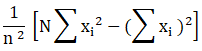= (1/102)[(10× 26360) – 5102]

= (1/100) (263600 –260100)

= 3500/100

= 35

WKT Standard deviation= √variance

= √35

= 5.91

So, co-efficient ofvariation = (σ/ x̅) × 100

= (5.91/51) × 100

= 11.58

Now, we have tocalculate Mean for y,

Mean ȳ = ∑yi/n

Where, n = number ofterms

= 1050/10

= 105

Then, Variance for y =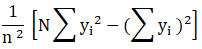= (1/102)[(10× 110290) – 10502]

= (1/100) (1102900 –1102500)

= 400/100

= 4

WKT Standard deviation= √variance

= √4

= 2

So, co-efficient ofvariation = (σ/ x̅) × 100

= (2/105) × 100

= 1.904

By comparing C.V. of Xand Y.

C.V of X > C.V. ofY

So, Y is more stablethan X.

Question 3 :

An analysis of monthly wages paid to workers in two firms A and B,belonging to the same industry, gives the following results:

 Firm A Firm B No. of wages earners 586 648 Mean of monthly wages Rs 5253 Rs 5253 Variance of the distribution of wages 100 121

(i) Which firm A or B pays larger amount as monthly wages?

(ii) Which firm, A or B, shows greater variability in individual wages?

(i) From the giventable,

Mean monthly wages offirm A = Rs 5253

and Number of wageearners = 586

Then,

Total amount paid =586 × 5253

= Rs 3078258

Mean monthly wages offirm B = Rs 5253

Number of wage earners= 648

Then,

Total amount paid =648 × 5253

= Rs 34,03,944

So, firm B pays largeramount as monthly wages.

(ii) Variance of firmA = 100

We know that, standarddeviation (σ)= √100

=10

Variance of firm B =121

Then,

Standard deviation(σ)=√(121 )

=11

Hence the standarddeviation is more in case of Firm B that means in firm B there is greatervariability in individual wages.

Question 4 :
The following is the record of goals scored by team A in a football session:
 No. of goals scored 0 1 2 3 4 No. of matches 1 9 7 5 3
For the team B, mean number of goals scored per match was 2 with a standard deviation 1.25 goals. Find which team may be considered more consistent?

From the given data,

Let us make the tableof the given data and append other columns after calculations.

 Number of goals scored xi Number of matches fi fixi Xi2 fixi2 0 1 0 0 0 1 9 9 1 9 2 7 14 4 28 3 5 15 9 45 4 3 12 16 48 Total 25 50 130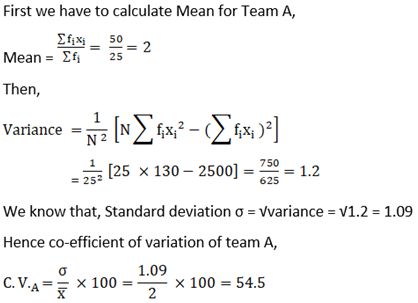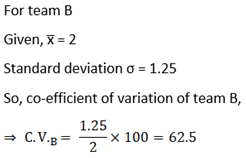Since C.V. of firm Bis greater

Team A is moreconsistent.

Question 5 :
The sum and sum of squares corresponding to length x (in cm) and weight y (in gm) of 50 plant products are given below: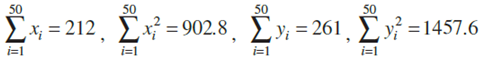Which is more varying, the length or weight?

First we have tocalculate Mean for Length x,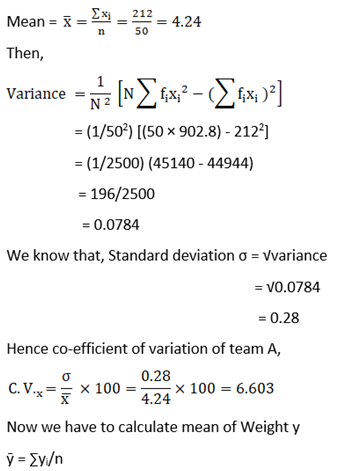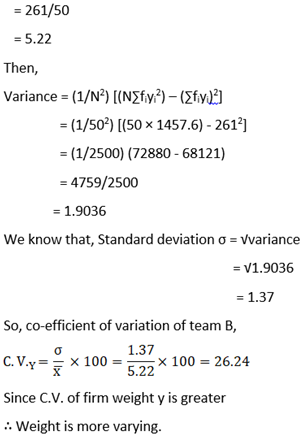Todays Deals### Chapter 15 Statistics Ex-15.3 Contributorskrishan

Name:
Email:

# Latest News# 9000 interview questions in different categories